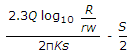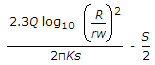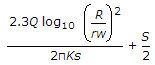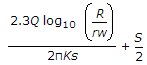# Online Civil Engineering Test - Water Supply Engineering TestLoading Test...

Instruction:

• This is a FREE online test. DO NOT pay money to anyone to attend this test.
• Total number of questions : 20.
• Time alloted : 30 minutes.
• Each question carry 1 mark, no negative marks.
• DO NOT refresh the page.
• All the best :-).

1.

Pick up the correct statement from the following :

A.
 Detention period for plain sedimentation tanks ranges between 4 to 8 hoursB.
 Detention period for sedimentation tanks, using coagulants usually ranges between 2 to 4 hoursC.
 The horizontally flow velocity in sedimentation tanks, is generally limited to 0.3 m/minuteD.
 All the above.2.

Corrosion of well pipes may not be reduced by

A.
 reducing the draw down and the pumping rateB.
 reducing the flow velocityC.
 using thicker pipesD.
 using screens having larger area of openingsE.
 none of these.3.

Turbidity of raw water is a measure of

A.
 suspended solidsB.
 acidity of waterC.
 B.O.D.D.
 none of these.4.

Increase in population of a rapidly growing city, may be estimated by

A.
 arithmetical mean methodB.
 geometrical methodC.
 incremental increase methodD.
 graphical comparison method.5.

Flow through period, in sedimentation tanks, is

A.
 equal to detention periodB.
 more than detention periodC.
 less than detention periodD.
 detention period divided by displacement efficiencyE.
 none of these.6.

The cast iron pipes for water supply system are used for

A.
 durabilityB.
 strengthC.
 easy connectionD.
 low maintenance costE.
 all the above.7.

In pumping stations, the type of joint generally used, is

A.
 socket and spigot jointB.
 flanged jointC.
 expansion jointD.
 dresser coupling jointE.
 flexible joint.8.

Maximum threshold number permitted for indicating the odour of public water supplies, is

A.
 1B.
 2C.
 3D.
 4E.
 59.

The approximate diameter of a water mains for supplying 7.2 mld with a velocity 1.2 m/sec, is

A.
 24 cmB.
 26 cmC.
 28 cmD.
 30 cmE.
 32 cm.10.

'Shrouding' is essentially provided in

A.
 strainer type wellsB.
 cavity type wellsC.
 slotted type wellD.
 all the above.11.

Chemical coagulation of drinking water, is done

A.
 to settle suspended materialsB.
 to increase rate of settlement of suspended materialsC.
 to remove the bacteriasD.
 none of these.12.

Dissolved carbon dioxide, can be removed from the supply main by

A.
 sedimentationB.
 aerationC.
 chlorinationD.
 coagulationE.
 none of these.13.

Q is the discharge from an unconfined tube well with depression head S through a pipe of radius rw. If the radius of influence is R, the minimum required length of the strainer, is

A.B.C.D.14.

Manholes are less common in

A.
 cast iron pipesB.
 steel pipesC.
 hume steel pipesD.
 R.C.C. pipes.15.

While designing a water supply of an industrial township, industrial and commercial water demand of total supply, is assumed

A.
 10%B.
 10 to 15%C.
 15 to 20%D.
 20 to 25%.16.

The flow of water gets retarded, in

A.
 settling tankB.
 sedimentation tankC.
 clariferD.
 sedimentation basinE.
 all the above.17.

Pick up the correct statement from the following :

A.
 The precipitation during its travel in atmosphere dissolves certain gasesB.
 Rain water which percolates through the ground, is free from suspended materialsC.
 Under ground water may dissolve minerals and salts present in the earth's layersD.
 Small quantities of iron, calcium and magnesium dissolved in water, may be useful for human healthE.
 All the above.18.

To pump water from a water reservoir 3 m deep and maximum water level at 135 m, a pump is installed to lift water up to R.L. 175 m at a constant rate of 36, 00, 000 litres per hour. If the length of the pipe is 1506 m and f = 0.01, ignoring other minor losses and assuming the economical diameter from Lea's formula D = 1.2 Q, the water horse power of the pump is

A.
 400B.
 450C.
 500D.
 580E.
 60019.

Most important method for calculating discharge for planning a water supply project, is

A.
 velocity area methodB.
 weir or spillway methodC.
 use of venturi-meterD.
 using power plant consumptionE.
 none of these.20.

Cast iron pipes

A.
 are widely used in city water suppliesB.
 resist corrosion satisfactorilyC.
 may last for 100 yearsD.
 are normally manufactured in lengths of about 3.5 mE.
 all the above.